Which of the following transformation least energy is required?

(a)                   (b) ${{P}^{-}}_{\left(g\right)}\to {P}_{\left(g\right)}+{e}^{-}$

(c) ${{S}^{-}}_{\left(g\right)}\to {S}_{\left(g\right)}+{e}^{-}$                    (d) $C{{l}^{-}}_{\left(g\right)}\to C{l}_{\left(g\right)}+{e}^{-}$

Concept Questions :-

Electron Affinity (EA)
High Yielding Test Series + Question Bank - NEET 2020

Difficulty Level:

Which of the following represents correct order of electron affinity?

(a) Cl>F>S>O                            (b) F>O>S>Cl

(c) F>Cl>S>O                            (d) Cl>S>O>F

Concept Questions :-

Electron Affinity (EA)
High Yielding Test Series + Question Bank - NEET 2020

Difficulty Level:

Correct expression of "Allred and Rochow's" scale is:

(a) Electronegativity =$0.744\frac{{Z}_{eff.}}{{r}^{2}}+0.359$

(b) Electronegativity =$0.359\frac{{r}^{2}}{{Z}_{eff.}}+0.744$

(c) Electronegativity =$0.359\frac{{Z}_{eff.}}{r}+0.744$

(d) Electronegativity =$0.359\frac{{Z}_{eff.}}{{r}^{2}}+0.744$

Concept Questions :-

Electronegativity
High Yielding Test Series + Question Bank - NEET 2020

Difficulty Level:

The process requiring absorption of energy is:

(a) $N\to {N}^{-}$                       (b) $F\to {F}^{-}$

(c) $Cl\to C{l}^{-}$                      (d) $H\to {H}^{-}$

Concept Questions :-

Electron Affinity (EA)
High Yielding Test Series + Question Bank - NEET 2020

Difficulty Level:

Which of the following compounds has a positive enthalpy of solution?

(a) LiF                                         (b) LiCl

(c) LiBr                                        (d) Lil

Concept Questions :-

Lattice/Hydration Energy
High Yielding Test Series + Question Bank - NEET 2020

Difficulty Level:

Which of the following compound is most acidic?

(a) $C{l}_{2}{O}_{7}$                              (b) ${P}_{4}{O}_{10}$

(c) $S{O}_{3}$                                 (d) ${B}_{2}{O}_{3}$

Concept Questions :-

Nature of Compound
High Yielding Test Series + Question Bank - NEET 2020

Difficulty Level:

If P and T are second period p-block elements then which of the following graph show correct relation between valence electrons in ${P}_{2}$ to ${T}_{2}$ (corresponding molecules) and their bond order is :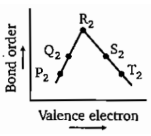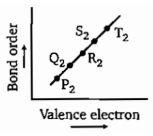(a)                                                                                    (b)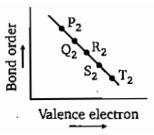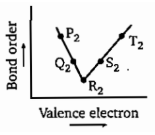(c)                                                                                     (d)

Concept Questions :-

Modern Periodic Table
High Yielding Test Series + Question Bank - NEET 2020

Difficulty Level:

A compound contains three elements A, B and C, if the oxidation number of A =+2, B =+5 and C =-2, the possible formula of the compound is :

(a) ${A}_{3}{\left({B}_{4}C\right)}_{2}$                                  (b) ${A}_{3}{\left(B{C}_{4}\right)}_{2}$

(c) ${A}_{2}{\left(B{C}_{3}\right)}_{2}$                                  (d) $AB{C}_{2}$

Concept Questions :-

Nature of Compound
High Yielding Test Series + Question Bank - NEET 2020

Difficulty Level:

Consider the following four elements, which are represented according to long form of periodic table.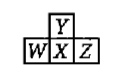Here W, Y and Z are left, up and right elements with respect to the element 'X' and 'X' belongs to 16th group and 3rd period.Then according to given information the incorrect statement regarding given elements is:

(a) Maximum electronegativity :Y              (b) Maximum catenation property :X

(c) Maximum electron affinity :Z               (d) Y exhibits variable covalency

Concept Questions :-

Electron Affinity (EA)
High Yielding Test Series + Question Bank - NEET 2020

Difficulty Level:

The ground state electronic configurations of the elements, U,V,W,X,and Y (these symbols do not have any chemical significance) are as follows:

Determine which sequence of elements satisfy the following statements :

(i) Element forms a carbonate which is not decomposed by heating

(ii) Element is most likely to form coloured ionic compounds

(iii) Element has largest atomic radius

(iv) Element forms only acidic oxide

(a) V W Y U                                   (b) V X Y W

(b) V W Y X                                   (d) V X W U

Concept Questions :-

Electronic Configuration